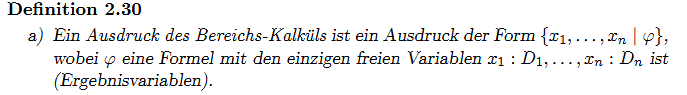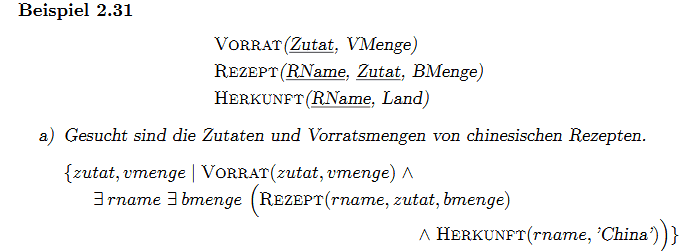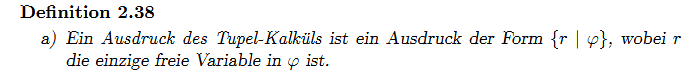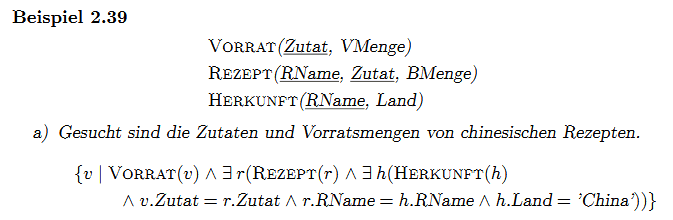# Relation between Sets AUFTRAG & KUNDE

• MHB
• mathmari
In summary, the conversation discusses relations and sets in the context of AUFTRAG and KUNDE. In order to determine certain sets, the concept of union and cartesian product are used. The conversation also touches on the first, third, and fourth property of these sets. Furthermore, the concept of intersection is mentioned, as well as the use of the symbol $\sigma_{condition}$ to select specific elements. Finally, there is a discussion about finding a subset of elements that contain the city "Gießen" in the last component.
mathmari said:
2 : We have written this with the expression $\pi_{\text{NAME}}(\text{KUNDE} \ltimes \text{AUFTRAG})$ which can be written in relational calculus with the expression $\{y \mid \exists x_1, x_2, x_3\left (\text{KUNDE} \ltimes \text{AUFTRAG}(x_1, x_2, x_3)\land y_1=x_1\land y_2=x_3\right \}$, right? Now we have to write also the left join in relational calculus, or not? :unsure:

Do we write $\text{KUNDE} \ltimes \text{AUFTRAG}(x_1, x_2, x_3)$ in relational calculus as $$\{y_1,y_2,y_3 \mid \exists x_1,x_2,x_3 \left [\text{KUNDE}(y_1,y_2, y_3) \land \left (\text{AUFTRAG}(x_1, x_2, x_3)\land \left (y_1=x_1\lor y_2=x_2\lor y_3=x_3\right )\right )\right ]\}$$ or how can we write that there must be a common column in the two tables? :unsure:

mathmari said:
As for the tuple calculus I found ine the notes the below example :

So at 1 we have then $$\{(k.KUNDNR, k.ORT)\mid \left (\text{KUNDE}(k)\right \}$$ or not?
Yep. Looks correct to me. (Nod)
You do have a superfluous parenthesis open before KUNDE though.

mathmari said:
Do we write $\text{KUNDE} \ltimes \text{AUFTRAG}(x_1, x_2, x_3)$ in relational calculus as $$\{y_1,y_2,y_3 \mid \exists x_1,x_2,x_3 \left [\text{KUNDE}(y_1,y_2, y_3) \land \left (\text{AUFTRAG}(x_1, x_2, x_3)\land \left (y_1=x_1\lor y_2=x_2\lor y_3=x_3\right )\right )\right ]\}$$ or how can we write that there must be a common column in the two tables?

I believe you already have it in relational calculus. That is, if I understand correctly what is meant by "relational calculus".
Perhaps you meant in tuple calculus? (Wondering)

If so, then following the example that you gave, I believe we can make it:
$$\{(k.NAME) \mid \text{KUNDE}(k) \land \exists a(\text{AUFTRAG}(a)\land k.KUNDNR=a.KUNDNR)\}$$Klaas van Aarsen said:
I believe you already have it in relational calculus. That is, if I understand correctly what is meant by "relational calculus".
Perhaps you meant in tuple calculus? (Wondering)

Ah can we use $\text{KUNDE} \ltimes \text{AUFTRAG}$ in the relational calculus? Do we nont have to write this left join also in relational calculus? Or is this allowed to use that? :unsure:

mathmari said:
Ah can we use $\text{KUNDE} \ltimes \text{AUFTRAG}$ in the relational calculus? Do we nont have to write this left join also in relational calculus? Or is this allowed to use that?
I guess we have to find the definitions of relational calculus and tuple calculus, and perhaps some examples.
Do you perchance have notes on them somewhere? (Wondering)

I found relational algebra that shows sets with tuples and also the use of e.g. $\text{KUNDE} \ltimes \text{AUFTRAG}$.Klaas van Aarsen said:
I guess we have to find the definitions of relational calculus and tuple calculus, and perhaps some examples.
Do you perchance have notes on them somewhere? (Wondering)

I found relational algebra that shows sets with tuples and also the use of e.g. $\text{KUNDE} \ltimes \text{AUFTRAG}$.I am looking these notes (they are in german). From pdf-page 20 we have relational calculus and from pdf-page 23 we have tuple calculus.mathmari said:
I am looking these notes (they are in german). From pdf-page 20 we have relational calculus and from pdf-page 23 we have tuple calculus.It says that the following is "Bereichs-Kalkül", which I think means Domain Calculus.And it says that the following is tuple calculus.It doesn't seem to refer to joins and such ($\Join$), so perhaps those are not intended to be used.

Either way, I guess we need to express each problem both as Domain Calculus and as Tuple Calculus.So do we have the following ?

1. Create an expression that lists all possible combinations of customer numbers (KUNDNR) and locations (ORT).

Domain Calculus : $\{kundnr, ort\mid \exists name\left (\text{KUNDE}(kundnr, name, ort)\right \}$
Tuple calculus : $\{(k.KUNDNR, k.ORT)\mid \left (\text{KUNDE}(k)\right \}$

2. Create an expression that lists all customer names (NAME) with at least one order (Auftrag).

Domain Calculus : $\{name \mid \exists auftragnr, datum, kundnr \left [\text{KUNDE}(kundnr, name, ort) \land \left (\text{AUFTRAG}(auftragng,datum, kundnr)\right )\right ]\}$
Tuple calculus : $\{(k.NAME) \mid \text{KUNDE}(k) \land \exists a(\text{AUFTRAG}(a)\land k.KUNDNR=a.KUNDNR)\}$

3. Create an expression that lists all order numbers (AUFTRAGNR) with customers from Gießen or Wetzlar.

Domain Calculus : $\{auftragnr \mid \exists kundnr \left [\text{KUNDE}(kundnr, name, ort) \land \left (\text{AUFTRAG}(auftragng,datum, kundnr)\right )\right ]\}$
Tuple calculus : $\{(a.AUFTRAGNR) \mid \text{AUFTRAG}(a) \land \exists k(\text{KUNDE}(k)\land k.KUNDNR=a.KUNDNR\land \left (k.ORT=\text{Gießen} \lor k.ORT=\text{Wetzlar}\right ))\}$

4. Create an expression that lists all customer numbers (KUNDNR) without an order (Auftrag).

Domain Calculus : $\{kundnr \mid \nexists auftragnr \left [\text{KUNDE}(kundnr, name, ort) \land \left (\text{AUFTRAG}(auftragng,datum, kundnr)\right )\right ]\}$
Tuple calculus : $\{(k.KUNDNR) \mid \text{KUNDE}(k) \land \exists a(k.KUNDNR\neq a.KUNDNR)\}$

5. Create an expression that lists all customer numbers (KUNDNR) that are not assigned to any order (Auftrag) and all customer numbers (KUNDNR) to which no name (NAME) is assigned.

Domain Calculus : $\{kundnr \mid\nexists auftragnr \left [\text{KUNDE}(kundnr, name, ort) \land \left (\text{AUFTRAG}(auftragng,datum, kundnr)\right )\right ] \land \nexists name \left [\text{KUNDE}(kundnr, name, ort) \right ]\}$
Tuple calculus : $\{(k.KUNDNR) \mid \text{KUNDE}(k) \land \exists a(k.KUNDNR\neq a.KUNDNR)\}$ How do we write the second condition?

:unsure:

mathmari said:
3. Create an expression that lists all order numbers (AUFTRAGNR) with customers from Gießen or Wetzlar.

Domain Calculus : $\{auftragnr \mid \exists kundnr \left [\text{KUNDE}(kundnr, name, ort) \land \left (\text{AUFTRAG}(auftragng,datum, kundnr)\right )\right ]\}$
Tuple calculus : $\{(a.AUFTRAGNR) \mid \text{AUFTRAG}(a) \land \exists k(\text{KUNDE}(k)\land k.KUNDNR=a.KUNDNR\land \left (k.ORT=\text{Gießen} \lor k.ORT=\text{Wetzlar}\right ))\}$

I'm missing reference to the $\text{Ort}$ in the Domain Calculus version. (Worried)

mathmari said:
4. Create an expression that lists all customer numbers (KUNDNR) without an order (Auftrag).

Domain Calculus : $\{kundnr \mid \nexists auftragnr \left [\text{KUNDE}(kundnr, name, ort) \land \left (\text{AUFTRAG}(auftragng,datum, kundnr)\right )\right ]\}$
Tuple calculus : $\{(k.KUNDNR) \mid \text{KUNDE}(k) \land \exists a(k.KUNDNR\neq a.KUNDNR)\}$

There seems to be an unbound $datum$ in the Domain Calculus version. (Worried)

In the tuple calculus version, won't there usually always be some order that is for a different client so that the condition is always satisfied when it shouldn't be? (Shake)

mathmari said:
5. Create an expression that lists all customer numbers (KUNDNR) that are not assigned to any order (Auftrag) and all customer numbers (KUNDNR) to which no name (NAME) is assigned.

Domain Calculus : $\{kundnr \mid\nexists auftragnr \left [\text{KUNDE}(kundnr, name, ort) \land \left (\text{AUFTRAG}(auftragng,datum, kundnr)\right )\right ] \land \nexists name \left [\text{KUNDE}(kundnr, name, ort) \right ]\}$
Tuple calculus : $\{(k.KUNDNR) \mid \text{KUNDE}(k) \land \exists a(k.KUNDNR\neq a.KUNDNR)\}$ How do we write the second condition?

I think we need the union of the customers without order, combined with the customers for which no name is available.
In other words, they should be combined with a logical or $(\lor)$ instead of a logical and $(\land)$.Furthermore, there are a couple of unbound variables, and the definition requires that there are none. (Worried)

The tuple calculus version can be written exactly the same as the domain calculus version - just with a different notation.3.Give an expression that lists all order numbers (AUFTRAGNR) with customers from Gießen or Wetzlar.

Domain Calculus : $$\{auftragnr \mid \exists kundnr ,name , ort,datum \left [\text{KUNDE}(kundnr, name, ort) \land \text{AUFTRAG}(auftragnr,datum, kundnr)\land \left (ort =\text{Gießen}\lor ort =\text{Wetzlar}\right )\right ]\}$$
Tuple calculus : $$\{(a.AUFTRAGNR) \mid \text{AUFTRAG}(a) \land \exists k(\text{KUNDE}(k)\land k.KUNDNR=a.KUNDNR\land \left (k.ORT=\text{Gießen} \lor k.ORT=\text{Wetzlar}\right ))\}$$

4. Create an expression that lists all customer numbers (KUNDNR) without an order (Auftrag).

Domain Calculus : $$\{kundnr \mid \exists auftragnr, name, ort, datum \left [\text{KUNDE}(kundnr, name, ort) \land \neg \left (\text{AUFTRAG}(auftragng,datum, kundnr)\right )\right ]\}$$
Tuple calculus : $$\{(k.KUNDNR) \mid \text{KUNDE}(k) \land \forall a(k.KUNDNR\neq a.KUNDNR)\}$$

5. Create an expression that lists all customer numbers (KUNDNR) that are not assigned to any order (Auftrag) and all customer numbers (KUNDNR) to which no name (NAME) is assigned.

Domain Calculus : $$\{kundnr \mid\exists name, ort, auftragnr, datum \left [\text{KUNDE}(kundnr, name, ort) \land \left (\neg\text{AUFTRAG}(auftragng,datum, kundnr) \lor \neg \text{KUNDE}(kundnr, name, ort)\right ) \right ]\}$$
Tuple calculus : $$\{(k.KUNDNR, a.KUNDNR) \mid \left [\text{KUNDE}(k) \land (k.KUNDNR\neq a.KUNDNR)\right ] \lor\left [ AUFTRAG(a) \land \exists k(a.KUNDNR\neq a. KUNDNR)\right ] \}$$

Are the expressions now correct? :unsure:

Last edited by a moderator:
mathmari said:
4. Create an expression that lists all customer numbers (KUNDNR) without an order (Auftrag).

Domain Calculus : $$\{kundnr \mid \exists auftragnr, name, ort, datum \left [\text{KUNDE}(kundnr, name, ort) \land \neg \left (\text{AUFTRAG}(auftragng,datum, kundnr)\right )\right ]\}$$
Suppose we have KUNDE(1, 'Ernst', 'Wetzlar') and AUFTRAG(10, 2021.01.01, 1) and also AUFTRAG(11, 2021.01.01, 2).
Then there exists an Auftrag that does not match the Kunde, and we find 'Ernst' even though he does have an Auftrag. (Shake)

mathmari said:
Tuple calculus : $$\{(k.KUNDNR) \mid \text{KUNDE}(k) \land \forall a(k.KUNDNR\neq a.KUNDNR)\}$$
Where does $a$ come from? (Wondering)

Klaas van Aarsen said:
Suppose we have KUNDE(1, 'Ernst', 'Wetzlar') and AUFTRAG(10, 2021.01.01, 1) and also AUFTRAG(11, 2021.01.01, 2).
Then there exists an Auftrag that does not match the Kunde, and we find 'Ernst' even though he does have an Auftrag. (Shake)

Should it be $\{kundnr \mid \exists name, ort \ \forall auftragnr, datum \left [\text{KUNDE}(kundnr, name, ort) \land \neg \left (\text{AUFTRAG}(auftragng,datum, kundnr)\right )\right ]\}$ ? :unsure:

Klaas van Aarsen said:
Where does $a$ come from? (Wondering)

Ah it should be $\{(k.KUNDNR) \mid \text{KUNDE}(k) \land \forall a(AUFTRAG(a)\land k.KUNDNR\neq a.KUNDNR)\}$,or not? :unsure:

mathmari said:
Should it be $\{kundnr \mid \exists name, ort \ \forall auftragnr, datum \left [\text{KUNDE}(kundnr, name, ort) \land \neg \left (\text{AUFTRAG}(auftragng,datum, kundnr)\right )\right ]\}$ ?

Ah it should be $\{(k.KUNDNR) \mid \text{KUNDE}(k) \land \forall a(AUFTRAG(a)\land k.KUNDNR\neq a.KUNDNR)\}$,or not?
Yep. (Nod)

And we had the same problem in question 5.Klaas van Aarsen said:
And we had the same problem in question 5.So we we have in question 5 the following ?

Domain Calculus : \begin{align*}\{&kundnr \mid \\ &\exists name, ort \ \forall auftragnr, datum \left [\text{KUNDE}(kundnr, name, ort) \land \neg\text{AUFTRAG}(auftragnr,datum, kundnr)\right ] \\ & \lor \exists auftragnr, datum \ \forall name, ort \left [\text{AUFTRAG}(auftragnr,datum, kundnr)\land \neg \text{KUNDE}(kundnr, name, ort)\right ) ]\}\end{align*}
Tuple Calculus : \begin{align*}\{(k.KUNDNR, a.KUNDNR) &\mid \left [\text{KUNDE}(k) \land \forall a(AUFTRAG(a) \land k.KUNDNR\neq a.KUNDNR)\right ] \\ & \lor\left [ AUFTRAG(a) \land \land \forall k(KUNDE(k)(a.KUNDNR\neq a. KUNDNR)\right ] \}\end{align*}

:unsure:

mathmari said:
So we we have in question 5 the following ?

Tuple Calculus : \begin{align*}\{(k.KUNDNR, a.KUNDNR) &\mid \left [\text{KUNDE}(k) \land \forall a(AUFTRAG(a) \land k.KUNDNR\neq a.KUNDNR)\right ] \\ & \lor\left [ AUFTRAG(a) \land \land \forall k(KUNDE(k)(a.KUNDNR\neq a. KUNDNR)\right ] \}\end{align*}
The result would consist of tuples like $\{ (1,1), (1,3), \ldots\}$. But shouldn't it be a set of KUNDNR like $\{1,3,\ldots\}$?Which $a.KUNDNR$ do we even have in the first part?There also seem to be a couple of typos in the last part of the expression. (Worried)

Klaas van Aarsen said:
The result would consist of tuples like $\{ (1,1), (1,3), \ldots\}$. But shouldn't it be a set of KUNDNR like $\{1,3,\ldots\}$?Which $a.KUNDNR$ do we even have in the first part?There also seem to be a couple of typos in the last part of the expression. (Worried)

Should it maybe be as follows?
\begin{align*}\{(k.KUNDNR) &\mid \left [\text{KUNDE}(k) \land \forall a(AUFTRAG(a) \land k.KUNDNR\neq a.KUNDNR)\right ] \\ & \lor\left [ \exists a \ AUFTRAG(a) \land \forall k\ KUNDE(k)\
(k.KUNDNR\neq a. KUNDNR)\right ] \}\end{align*}

:unsure:

mathmari said:
Should it maybe be as follows?
\begin{align*}\{(k.KUNDNR) &\mid \left [\text{KUNDE}(k) \land \forall a(AUFTRAG(a) \land k.KUNDNR\neq a.KUNDNR)\right ] \\ & \lor\left [ \exists a \ AUFTRAG(a) \land \forall k\ KUNDE(k)\
(k.KUNDNR\neq a. KUNDNR)\right ] \}\end{align*}
Doesn't the $k.KUNDNR$ only apply to the first half to the expression?
The second half has a $\forall k$, so it does not tie to any particular $k.KUNDNR$ does it? (Worried)

Klaas van Aarsen said:
Doesn't the $k.KUNDNR$ only apply to the first half to the expression?
The second half has a $\forall k$, so it does not tie to any particular $k.KUNDNR$ does it? (Worried)

Ok... But how can we write this then? At the second part we want to check if the KUNDNR of Auftrag is nont included in KUNDE, or not? I got stuck right now. :unsure:

mathmari said:
Ok... But how can we write this then? At the second part we want to check if the KUNDNR of Auftrag is nont included in KUNDE, or not? I got stuck right now.
\begin{align*}\{KUNDNR &\mid \exists k\left (\text{KUNDE}(k)
\land k.KUNDNR=KUNDNR \land \ldots\right)\lor
\exists a\left (\text{AUFTRAG}(a)
\land a.KUNDNR=KUNDNR \land \ldots\right)
\}\end{align*}Klaas van Aarsen said:
\begin{align*}\{KUNDNR &\mid \exists k\left (\text{KUNDE}(k)
\land k.KUNDNR=KUNDNR \land \ldots\right)\lor
\exists a\left (\text{AUFTRAG}(a)
\land a.KUNDNR=KUNDNR \land \ldots\right)
\}\end{align*}Ah you mean :
\begin{align*}\{KUNDNR \mid & \exists k\left (\text{KUNDE}(k)
\land k.KUNDNR=KUNDNR \land \forall a(AUFTRAG(a) \land k.KUNDNR\neq a.KUNDNR)\right)\\ &\lor
\exists a\left (\text{AUFTRAG}(a)
\land a.KUNDNR=KUNDNR \land \forall k\ KUNDE(k)\
(k.KUNDNR\neq a. KUNDNR)\right)
\}\end{align*} right? :unsure:

Yep. (Sun)

We consider the relations AUFTRAG and KUNDE and create an SQL expression for each of the below.

1. Create an expression that lists all possible combinations of customer numbers and locations related to KUNDE.
2. Create an expression that lists all customer names with at least one order.
3. Create an expression that lists all order numbers with customers from Gießen or Wetzlar.
4. Create an expression that lists all customer numbers without an order.

I have done the following :

Expression for 1 :
SELECT DISTINCT KUNDNR, ORT
FROM KUNDE

Expression for 2 :
SELECT DISTINCT NAME
FROM KUNDE
WHERE k.KUNDNR = a.KUNDNR

Expression for 3 :
SELECT DISTINCT AUFTRAGNR
FROM AUFTRAG
WHERE a.KUNDNR = k.KUNDNR AND (k.ORT = Gießen OR k.ORT = Wetzlar)

Expression for 4 :
SELECT DISTINCT KUNDNR
FROM KUNDE
WHERE k.KUNDNR != a.KUNDNR

Are the expressions correct? :unsure:

mathmari said:
1. Create an expression that lists all possible combinations of customer numbers and locations related to KUNDE.
Expression for 1 :
SELECT DISTINCT KUNDNR, ORT
FROM KUNDE

That will list the customers with the location that is registered for them. That would be 1 record for each customer.
However, it seems that the question asks for each possible combination of each existing customer with each existing location.mathmari said:
Expression for 2 :
SELECT DISTINCT NAME
FROM KUNDE
WHERE k.KUNDNR = a.KUNDNR

Expression for 3 :
SELECT DISTINCT AUFTRAGNR
FROM AUFTRAG
WHERE a.KUNDNR = k.KUNDNR AND (k.ORT = Gießen OR k.ORT = Wetzlar)

Expression for 4 :
SELECT DISTINCT KUNDNR
FROM KUNDE
WHERE k.KUNDNR != a.KUNDNR
The WHERE clause is a condition. Your WHERE clauses refer to k and a, but they are not defined anywhere. (Worried)

Klaas van Aarsen said:
That will list the customers with the location that is registered for them. That would be 1 record for each customer.
However, it seems that the question asks for each possible combination of each existing customer with each existing location.Ah ok.. So do we not have to use "DISTINCT" or just in an other way? :unsure:

Klaas van Aarsen said:
The WHERE clause is a condition. Your WHERE clauses refer to k and a, but they are not defined anywhere. (Worried)

Ah yes... So should it be as follows?

Expression for 2 :
SELECT DISTINCT NAME
FROM KUNDE k, AUFTRAG a
WHERE k.KUNDNR = a.KUNDNR

Expression for 3 :
SELECT DISTINCT AUFTRAGNR
FROM AUFTRAG a, KUNDE k
WHERE a.KUNDNR = k.KUNDNR AND (k.ORT = Gießen OR k.ORT = Wetzlar)

Expression for 4 :
SELECT DISTINCT KUNDNR
FROM KUNDE k, AUFTRAG a
WHERE k.KUNDNR != a.KUNDNR

:unsure:

It should be in another way - at least if I understand the question correctly.
They seem to be asking for a cartesian product.
Then again, it does seem to be a bit too advanced given the other questions. :unsure:

DISTINCT has no effect here. That is because KUNDNR is unique in KUNDE. It is a so called primary key.Questions 2 and 3 look fine now. (Nod)

For question 4 we will also get customers that do have an order. So that is not correct. (Shake)

Klaas van Aarsen said:
It should be in another way - at least if I understand the question correctly.
They seem to be asking for a cartesian product.
Then again, it does seem to be a bit too advanced given the other questions. :unsure:

DISTINCT has no effect here. That is because KUNDNR is unique in KUNDE. It is a so called primary key.Do we maybe write :

Expression for 1 :
SELECT KUNDE.kundnr, KUNDE.ort
FROM KUNDE

:unsure:

Klaas van Aarsen said:
Questions 2 and 3 look fine now. (Nod)

So we need in these cases "DISTINCT", right? :unsure:

Klaas van Aarsen said:
For question 4 we will also get customers that do have an order. So that is not correct. (Shake)

Ah we have to write that this inequality holds for each a in AUTRAG, don't we? :unsure:

mathmari said:
Do we maybe write :

Expression for 1 :
SELECT KUNDE.kundnr, KUNDE.ort
FROM KUNDE

That is effectively the same as before.

I think it might be:
SELECT k1.kundnr, k2.ort
FROM KUNDE k1, KUNDE k2
:unsure:

mathmari said:
So we need in these cases "DISTINCT", right?

In question 2, yes, we can expect that the same customer has multiple orders, so their name will get listed multiple times.
DISTINCT then ensures each name is listed only once.In question 3 it is not be necessary. Orders are unique. So if we list the AUFTRAGNR's, we will already have at most one of each.mathmari said:
Ah we have to write that this inequality holds for each a in AUTRAG, don't we?

Indeed. (Sweating)

Last edited:
Klaas van Aarsen said:
That is effectively the same as before.

I think it might be:
SELECT k1.kundnr, k2.ort
FROM KUNDE k1, KUNDE k2
:unsure:

So that we consider two different columns? :unsure:

Klaas van Aarsen said:
In question 2, yes, we can expect that the same customer has multiple orders, so their name will get listed multiple times.
DISTINCT then ensures each name is listed only once.In question 3 it is not be necessary. Orders are unique. So if we list the AUFTRAGNR's, we will already have at most one of each.Indeed. (Sweating)

So do we have to write the following ?

Expression for 1 :
SELECT k1.kundnr, k2.ort
FROM KUNDE k1, KUNDE k2

Expression for 2 :
SELECT DISTINCT NAME
FROM KUNDE k, AUFTRAG a
WHERE k.KUNDNR = a.KUNDNR

Expression for 3 :
SELECT DISTINCT AUFTRAGNR
FROM AUFTRAG a, KUNDE k
WHERE a.KUNDNR = k.KUNDNR AND (k.ORT = Gießen OR k.ORT = Wetzlar)

Expression for 4 :
SELECT DISTINCT KUNDNR
FROM KUNDE k
WHERE k.KUNDNR != ALL
$\ \ \ \ \$(SELECT KUNDNR
$\ \ \ \ \$FROM AUFTRAG)

:unsure:

Looks right to me. (Nod)